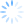Login/Register Math Worksheets, Activities, Games Helpline 099826-99306

Math worksheets on Fractions: Improper and Mixed fractions

Fraction worksheets for grade 4 and grade 5 student that you can download and print for free. Here you will find a fractions worksheet on mixed fractions and improper fractions concept.

As a concept fractions are often challenging and confusing for students. This is the first time when they are looking at this "number upon number" format of expressing information. We at BrainX make worksheets to either bring out applications of fractions or share the topics like a colorful math game that students love to play. The same applies for fractions that are introduced in grade 3, grade 4 and then built up in grade 5.

Fractions conversion from improper fractions to mixed fractions

Download this math fractions worksheet on mixed fractions and improper fractions. There is a specific arrow for each head of the ten headed Ravan. Point the arrows to the right head of Ravan by converting mixed fractions to improper fractions or vice versa. Use this colorful math activity sheet to kill the demon of fear of fractions among students of grade 4 and grade 5.• Worksheet focus

• Grade for this worksheet: Class 4 Maths or Class 5 maths
• Major focus of this worksheet: conversion of fractions from mixed to improper fractions and vice versa

You can find more such math worksheets for grade 4 and math worksheets for grade 5 as a part of BrainX Maths Training Program. More samples are uploaded every week. So keep checking out the BrainX samples page for new maths worksheets, games and real life applications for free.

This worksheet on fractions is of moderate level of difficulty for grade 4 and grade 5 students. The introductory activities on mixed and improper fractions start with pictoral representation of improper and mixed fractions that demonstrate the meaning of mixed fractions. Then the skill level is moved up to existence of mixed fractions in real life situations and then fraction worksheets focus on conversion of mixed fractions to improper and vice versa. More advanced fraction worksheets follow that involve rationalizing fractions, finding common denominator, addition, subtraction, multiplication and division of fractions too.  Conversion of fractions to decimals is addressed through interesting real life applications as well.